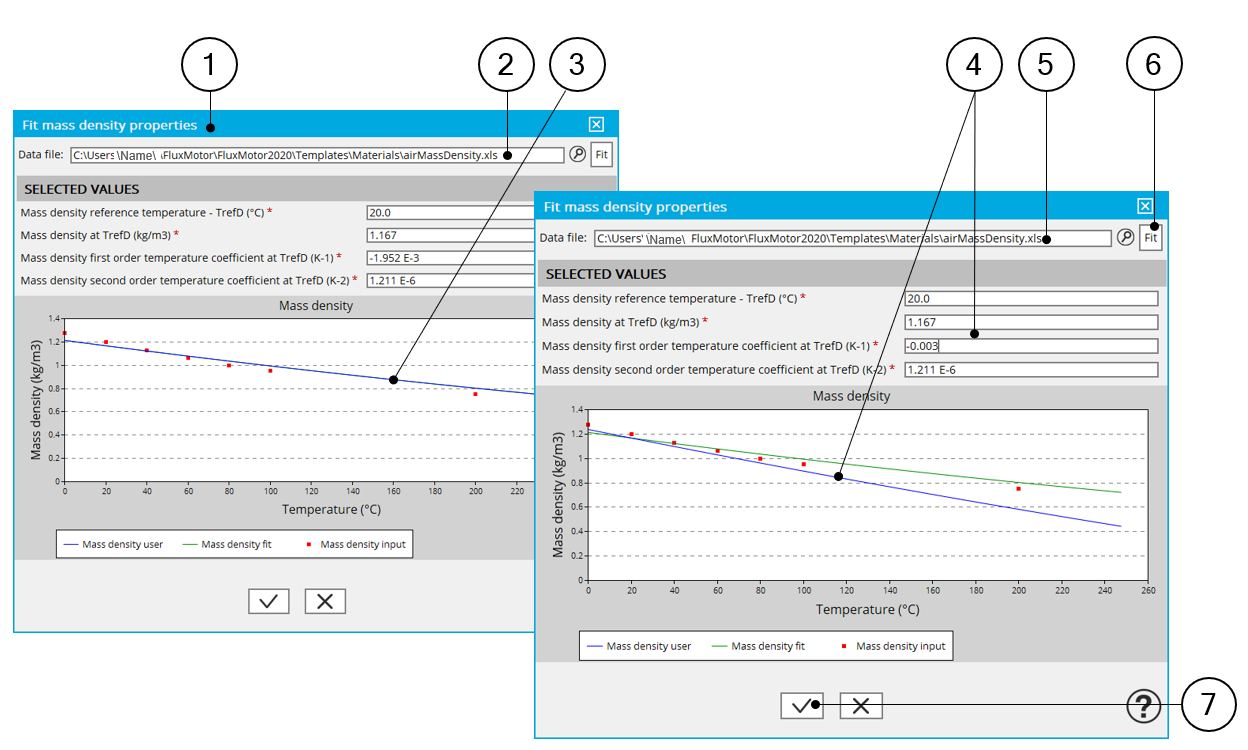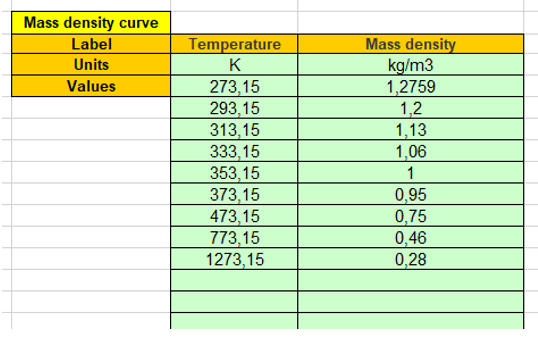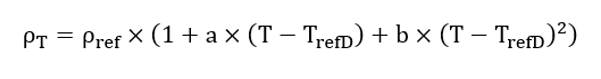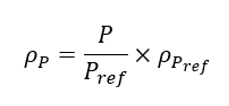# Definition of the gas mass density

## 1. Introduction - Reminder

Here is the process to define the gas thermal characteristics from the importation of series of points representing the considered quantity curve listed in an Excel file. In the following example air mass density is considered, however the same principle is applied for all other gas thermal quantity which are defined below.Identification of the air mass density curve characteristics (for instance) 1 Dialog box allowing the characterization of the density curve imported from an Excel file 2 Path where Excel file to be imported is stored. See an example of Excel file below. 3 When importing an Excel file, points representing the density curve are listed, an optimization process automatically computes and displays the corresponding characteristics. At the same time three curves are displayed: Red points are the imported points (listed in the Excel file) Green curve is the resulting curve computed by the optimization process. This corresponds to the computed characteristics and displayed just after the computation. The blue curve shows modifications induced when the characteristics are changed by the user. 4 Indeed, the user can adjust one or all the three main characteristics of the density curve. · The density at reference temperature · The density first order temperature coefficient · The density second order temperature coefficient 5 Field in which the path where the Excel file to be imported is stored. 6 At any time, the user can run the optimization process to get back the proposed values. 7 The last parameter values, written in the input fields are validated when the user clicks on this button. Validation of the last parameter values is achieved when clicking on this button. It is possible to cancel the creation of the density curve model. In this case, the previous values defined before opening this dialog box are reset.

Example of an Excel file to define the mass density curve parameters.Example of an Excel file to define the air mass density curve parameters

## 2. Mass densitySymbol Definition Unit P ref Reference pressure Pa T refD Mass density reference temperature T refD °C r ref Mass density at T refD and P ref kg/m3 a Mass density first order temperature coefficient at T refD and P ref K-1 b Mass density second order temperature coefficient at T refD and P ref K-2
Note:
1. The reference pressure mentioned in the previous table is also the one considered for defining the gas specific heat.
2. For a given temperature, the gas density (kg/m 3 ) changes with the pressure following the perfect gas law

The mass density ρ computed at a pressure P is computed as below: